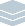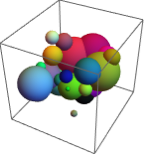Wolfram Archive
Wolfram Programming Lab is a legacy product.
All the same functionality and features, including access to Programming Lab Explorations, are available with Wolfram|One.
Start programming now. »
Try it now »
(no sign-in required)# Random Spheres

Make random arrangements of 3D spheres (a good model for many materials).

Run the code to draw 20 random spheres in a size-5 region. Try more spheres:

This draws a sphere at coordinate {0,0,0}:

Graphics3D[Sphere[{0, 0, 0}]]

Add PlotRange6 to make the drawing area extend 6 units in all directions, and AxesTrue to turn on axes so you can see the coordinate values. Rotate the graphic with the mouse to see it from all angles:

Graphics3D[Sphere[{0, 0, 0}], PlotRange -> 6, Axes -> True]

Use RandomReal to draw a sphere in a random position.

This gives a random number between 0 and 5. Every time you run the code, you get a different number:

RandomReal

This gives a list of three random numbers between 0 and 5. Every time you run the code, you get a different list:

RandomReal[5, 3]

Replace {0,0,0} with RandomReal[5,3] in the sphere-drawing code to draw the sphere in a different position every time you run the code:

Graphics3D[Sphere[RandomReal[5, 3]], PlotRange -> 6, Axes -> True]

Use Table to draw lots of random spheres.

Table makes lists of things, like this list of 20 random numbers:

Table[RandomReal, {20}]

This uses Table to draw 20 spheres in random positions:

Graphics3D[Table[Sphere[RandomReal[5, 3]], {20}], PlotRange -> 6, Axes -> True]

Turn off the axes by removing AxesTrue, and remove PlotRange6 too. Without an explicit PlotRange value, the plot range is calculated automatically to be just large enough to contain the contents of the graphic:

Graphics3D[Table[Sphere[RandomReal[5, 3]], {20}]]

Graphics3D[Table[Sphere[RandomReal[5, 3]], {20}]]

Give the spheres 50% opacity. Try opacities other than .5:

This draws an opaque sphere:

Graphics3D[Sphere[{0, 0, 0}]]

This draws a sphere that is 50% opaque (and thus 50% transparent):

Graphics3D[{Opacity[.5], Sphere[{0, 0, 0}]}]

Graphics3D[Table[{Opacity[.5], Sphere[RandomReal[5, 3]]}, {20}]]

Make an interactive version. Drag the seed slider for different arrangements:

Heres the code to draw 20 transparent spheres in random positions:

Graphics3D[Table[{Opacity[.5], Sphere[RandomReal[5, 3]]}, {20}]]

Use Manipulate to make the number of spheres interactive.

Wrap the expression with Manipulate[...], replace 30 with the variable number and specify that number ranges from 5 to 200 in steps of 1. Drag the slider to change the number of spheres:

Manipulate[ Graphics3D[ Table[{Opacity[.5], Sphere[RandomReal[5, 3]]}, {number}]], {number, 5, 200, 1} ]

The spheres jump around as you drag the slider because RandomReal gives different coordinate positions each time.

If you add SeedRandom to the code, the sphere arrangement stays the same as you drag the slider. Thats because SeedRandom resets the random number generator to the same point each time the spheres are drawn, so that you get the same sequence of random numbers:

Manipulate[ SeedRandom; Graphics3D[ Table[{Opacity[.5], Sphere[RandomReal[5, 3]]}, {number}]], {number, 5, 200, 1} ]

Let the random number seed vary from 10000 to 50000 in steps of 1 to select different sphere arrangements:

Manipulate[ SeedRandom[seed]; Graphics3D[ Table[{Opacity[.5], Sphere[RandomReal[5, 3]]}, {number}]], {number, 5, 200, 1}, {seed, 10000, 50000, 1} ]

Add a control to set the opacity. Let the opacity vary from 0 to 1 with an initial value of 1:

Manipulate[ SeedRandom[seed]; Graphics3D[ Table[{Opacity[opacity], Sphere[RandomReal[5, 3]]}, {number}]], {{opacity, 1}, 0, 1}, {number, 5, 200, 1}, {seed, 10000, 50000, 1} ]

Manipulate[ SeedRandom[seed]; Graphics3D[ Table[{Opacity[opacity], Sphere[RandomReal[5, 3]]}, {number}]], {{opacity, 1}, 0, 1}, {number, 5, 200, 1}, {seed, 10000, 50000, 1} ]

Use random colors for the spheres:

RandomColor does just what youd expect. You get a different color each time you run the code:

RandomColor[]

Add RandomColor to the sphere code to color the spheres randomly:

Manipulate[ SeedRandom[seed]; Graphics3D[ Table[{RandomColor[], Opacity[opacity], Sphere[RandomReal[5, 3]]}, {number}]], {{opacity, 1}, 0, 1}, {number, 5, 200, 1}, {seed, 10000, 50000, 1} ]

Manipulate[ SeedRandom[seed]; Graphics3D[ Table[{RandomColor[], Opacity[opacity], Sphere[RandomReal[5, 3]]}, {number}]], {{opacity, 1}, 0, 1}, {number, 5, 200, 1}, {seed, 10000, 50000, 1} ]

Allow random sphere sizes between 0 and 1.5. Try maximum sizes other than 1.5:

Add a second value to Sphere to specify its radius. This sphere has a radius of 1:

Graphics3D[Sphere[{0, 0, 0}, 1], PlotRange -> 2]

Replace 1 with RandomReal[1.5] to get a different radius between 0 and 1.5 each time you run the code:

Graphics3D[Sphere[{0, 0, 0}, RandomReal[1.5]], PlotRange -> 2]

Make the spheres in the interactive code random sizes by specifying a radius of RandomReal[1.5] in Sphere:

Manipulate[ SeedRandom[seed]; Graphics3D[ Table[{RandomColor[], Opacity[opacity], Sphere[RandomReal[5, 3], RandomReal[1.5]]}, {number}]], {{opacity, 1}, 0, 1}, {number, 5, 200, 1}, {seed, 10000, 50000, 1} ]

Manipulate[ SeedRandom[seed]; Graphics3D[ Table[{RandomColor[], Opacity[opacity], Sphere[RandomReal[5, 3], RandomReal[1.5]]}, {number}]], {{opacity, 1}, 0, 1}, {number, 5, 200, 1}, {seed, 10000, 50000, 1} ]

Constrain the range to be able to chop off the tops of spheres:

By default, a graphic is made just large enough to accommodate its contents:

Graphics3D[ Table[{RandomColor[], Sphere[RandomReal[5, 3], RandomReal[1.5]]}, {5}]]

You can specify a plot range to limit a graphics extent. This graphic ranges from 0 to 3 in the vertical (z) direction. Spheres that cross the plot range boundaries are chopped open:

Graphics3D[ Table[{RandomColor[], Sphere[RandomReal[5, 3], RandomReal[1.5]]}, {5}], PlotRange -> {0, 3}]

Add a plot range control to the interactive code so you can slice open the spheres:

Manipulate[ SeedRandom[seed]; Graphics3D[ Table[{RandomColor[], Opacity[opacity], Sphere[RandomReal[5, 3], RandomReal[1.5]]}, {number}], PlotRange -> {0, range}], {{opacity, 1}, 0, 1}, {number, 5, 200, 1}, {seed, 10000, 50000, 1}, {range, 3, 6} ]

Manipulate[ SeedRandom[seed]; Graphics3D[ Table[{RandomColor[], Opacity[opacity], Sphere[RandomReal[5, 3], RandomReal[1.5]]}, {number}], PlotRange -> {0, range}], {{opacity, 1}, 0, 1}, {number, 5, 200, 1}, {seed, 10000, 50000, 1}, {range, 3, 6} ]

Share Itmake an interactive sphere website:

Deploy the Manipulate to the Wolfram Cloud, where anyone with a browser can use it:

CloudDeploy[ Manipulate[ SeedRandom[seed]; Graphics3D[ Table[{RandomColor[], Opacity[opacity], Sphere[RandomReal[5, 3], RandomReal[1.5]]}, {number}], PlotRange -> {0, range}], {{opacity, 1}, 0, 1}, {number, 5, 200, 1}, {seed, 10000, 50000, 1}, {range, 3, 6} ], Permissions -> "Public" ]

Click the link in the output to visit the site.